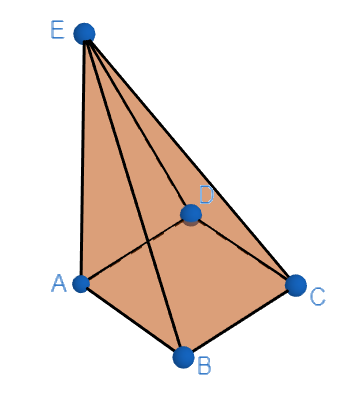Filters
Latest Questions
CBSE
Mathematics
Euler's form
If $z = 3 - 4i$ is turned ${90^0}$ in anti clock direction, then new position of $z$ is
A.$3 - 4i$
B.$4 - 3i$
C.$4 + 3i$
D.$3 + 4i$
CBSE
Mathematics
Euler's form
Find the real part of $z={{e}^{{{e}^{i\theta }}}}$, $\theta \in R$.

CBSE
Mathematics
Euler's form
If ${e^{i\theta }} = \cos \theta + i\sin \theta$ then in $\Delta ABC$ value of ${e^{iA}}.{e^{iB}}.{e^{iC}}$ is
A) $- i$
B) $1$
C) $- 1$
D) None of these
CBSE
Mathematics
Euler's form
The amplitude of $\sin \dfrac{\pi }{5} + i\left( {1 - \cos \dfrac{\pi }{5}} \right)$ is…
CBSE
Mathematics
Euler's form
Verify the Euler’s formula for the given solid.CBSE
Mathematics
Euler's form
What does $\dfrac{{{e}^{ix}}-{{e}^{-ix}}}{2i}$ equal?
CBSE
Mathematics
Euler's form
If $\Phi \left( N \right)$ is the number of integers which are less than $N$ and prime to it, and if $x$ is prime to $N,$ show that: ${{x}^{\Phi \left( N \right)}}-1\equiv 0\left( \bmod N \right).$
CBSE
Mathematics
Euler's form
How do you evaluate ${{e}^{\dfrac{\pi }{12}i}}-{{e}^{\dfrac{13\pi }{8}i}}$ using trigonometric functions?
CBSE
Mathematics
Euler's form
How do you graphically divide complex numbers?

CBSE
Mathematics
Euler's form
A root of ${x^5} - 32 = 0\;$ lies in quadrant II. Write this root in polar form.
$2\left( {\cos {{120}^0} + i\sin {{120}^0}} \right)$
A. $2\left( {\cos {{144}^0} + i\sin {{144}^0}} \right)$
B. $2\left( {\cos {{150}^0} + i\sin {{150}^0}} \right)$
C. $4\left( {\cos {{144}^0} + i\sin {{144}^0}} \right)$
D. $2\left( {\cos {{72}^0} + i\sin {{72}^0}} \right)$
CBSE
Mathematics
Euler's form
How does it relate $e$ to $\pi$ ?

CBSE
Mathematics
Euler's form
How many edges are there in a cuboid?
(A) $3$
(B) $4$
(C) $5$
(D) $6$
(E) $12$

Prev
1
2
3